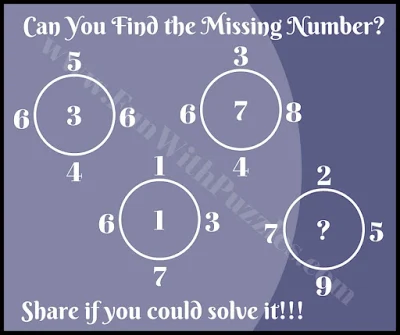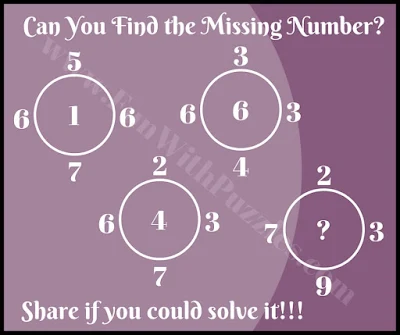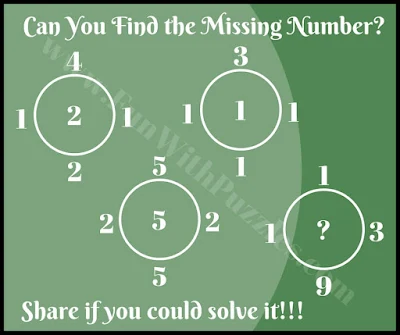We have earlier solve some Simple and Easy Circle Math Brain Teasers. Now it's time to solve some tricky Math Brain Puzzles. In the earlier post of Circle, Math Brain Teaser puzzles were very easy and can be solved by the kids without much effort. Now it is time to enhance the level of puzzles to make these puzzles tricky. So are you ready to take this tricky Maths Brain Challenge?
In these Maths Brain Challenges, you are given five, Circle Brain Teasers. In each of these brain teasers, there are four circles. Each of the circles is having five numbers with one number being inside the circle and the other four being outside the circle. You have to study the relationship between these numbers in each of the three numbers and then solve it for the 4th circle where one number is missing.
So are you ready to find the missing number in these brain teasers? These Math Brain Challenges starts with the easy puzzle to get you started. The remaining four brain teasers are tough and will tickle your mind. We are providing the answers to these puzzles at the end. Do write your answers with explanations in the comment section of this post.1. Easy maths brain picture puzzle challenge2. Brain teasing maths brain picture puzzle challenge3. Brain twisting maths brain picture puzzle challenge4. Tough maths brain picture puzzle challenge5. Tricky maths brain picture puzzle challenge
Answers to these math brain challenges are given below. Do use these answers only to validate your solutions and try to solve these brain teasers without looking at these answers.

A1. 1

A2. 3

A3. 2

A4. 8

A5. 5